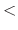Next: Grid Forces Up: User Defined Forces Previous: Constant Forces

## External Electric Field

NAMD provides the ability to apply a constant electric field to the molecular system being simulated. Energy due to the external field will be reported in the MISC column and will be continuous even in simulations using periodic boundary conditions as unwrapped coordinates are used to calculate energy and pressure, resulting in linearly increasing pressure over time for systems with free ions. To avoid this effect, for periodic simulations the new eFieldNormalized option should be used with the electric field vector multiplied by the cell dimension. There are three parameters that control this feature.

• eFieldOnapply electric field?Acceptable Values: yes or no
Default Value: no
Description: Specifies whether or not an electric field is applied.

• eFieldelectric field vectorAcceptable Values: vector of decimals (x y z)
Description: Vector which describes the electric field to be applied. Units are, which is natural for simulations. This parameter may be changed between run commands, allowing a square wave or other approximate wave form to be applied.

• eFieldNormalizedelectric field vector scaled by cell basis vectors?Acceptable Values: yes or no
Default Value: no
Description: Specifies whether or not that eField vector has been scaled by the cell basis vectors, thus indicating the voltage drop across the cell in units of. The eField vector is then scaled by the reciprocal lattice vectors at each timestep. When eFieldNormalized is true the eField forces do not contribute to the pressure calculation.Next: Grid Forces Up: User Defined Forces Previous: Constant Forces
http://www.ks.uiuc.edu/Research/namd/